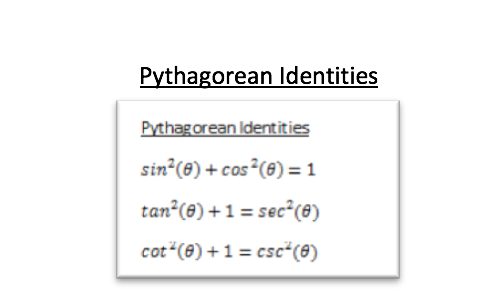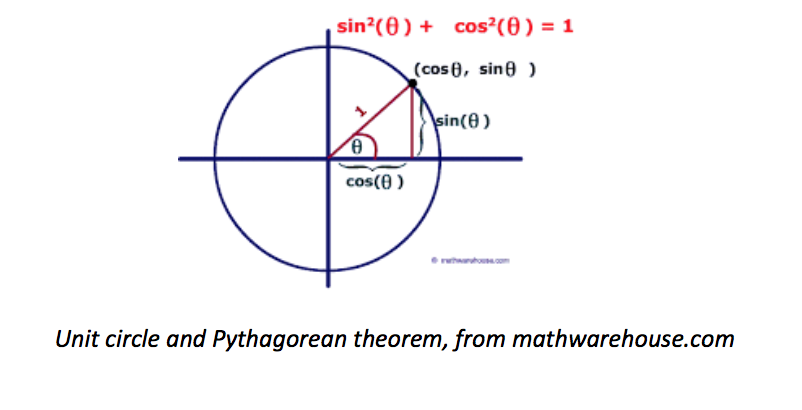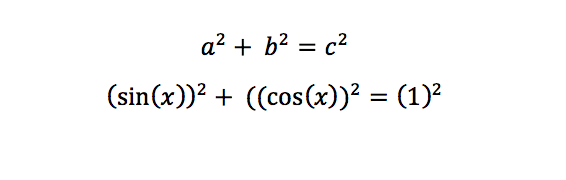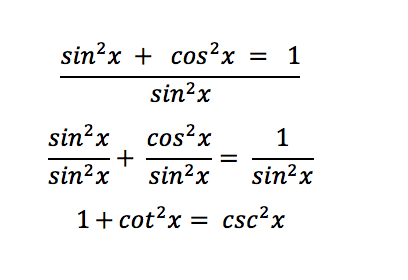# Trig Identities: Which Ones You Should, and Which Ones You Shouldn’t Memorize Part IIAs the name implies, the Pythagorean identities stem from the Pythagorean theorem (which you should already remember as a2 + b2 = c2).  The first of the three you should memorize, as it is the most common and useful.  However, it isn’t hard to derive where it came from using the unit circle:The unit circle is a circle with a radius of “1” centered at the origin.  We know that the cosine function will give us the x-coordinate of the point on a unit circle for each angle (e.g., the cosine of 90 degrees is zero since 90 degrees is the “top” of the circle which is on the y-axis).  We know that the sine function does the same for the y-coordinate of each angle on the unit circle.

Since the radius of the circle is always one, we can see in the image above that we can form a right triangle for any given angle where the radius is the hypotenuse.  Since we know the Pythagorean theorem gives us the length of the hypotenuse about the legs, we can substitute what we know into the theorem to get our new identity.This is why the primary Pythagorean identity works.  It is good to know, but you will still likely end up memorizing it anyway.  The next two Pythagorean identities, however, absolutely do not need to be memorized since they are essentially the same identity repeated.

Let’s see what happens if we take our main Pythagorean identity and simply divide everything by sin2(x):The first term simply becomes 1, the second term becomes cotangent from our tangent identity, and the final term becomes cosecant from our reciprocal identity.  Let’s do the same thing except we’ll divide by cos2(x):The first term becomes tangent from the tangent identity, the second term is just one, and the last term becomes secant from the reciprocal identity.

We now have both of our remaining Pythagorean identities, just from basic manipulations of the original.  We could construct similar identities by dividing by other terms.  We could also multiply by other terms.  Or add or subtract to both sides.  It is no different than saying that if x = a, then 2x = 2a.

Don’t memorize the last two of the Pythagorean identities; just know how to get to them and similar identities.  In trigonometry, it’s a skill you will need regardless.

Stay tuned for part III.

Our online math tutors are the most experienced in SoCal. Signing up is easy.

Michael C. is currently a private online math, science, and standardized test tutor with TutorNerd in Irvine and Anaheim.